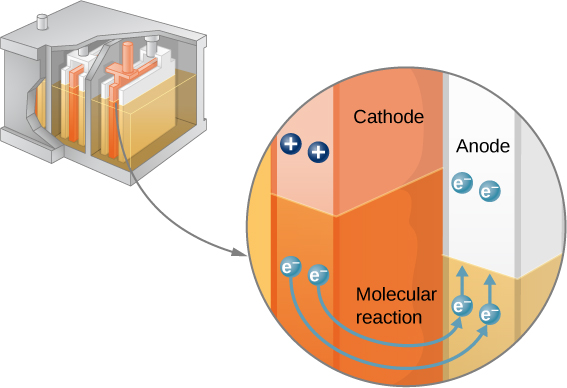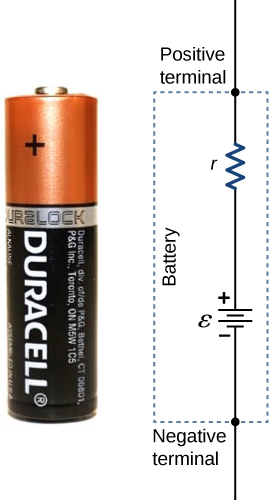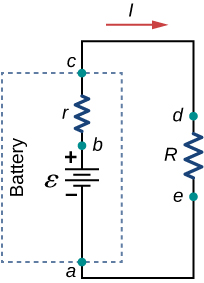# 10.1 Electromotive force  (Page 3/11)

 Page 3 / 11

Note that the reaction does not take place unless there is a complete circuit to allow two electrons to be supplied to the cathode. Under many circumstances, these electrons come from the anode, flow through a resistance, and return to the cathode. Note also that since the chemical reactions involve substances with resistance, it is not possible to create the emf without an internal resistance.In a lead-acid battery, two electrons are forced onto the anode of a cell, and two electrons are removed from the cathode of the cell. The chemical reaction in a lead-acid battery places two electrons on the anode and removes two from the cathode. It requires a closed circuit to proceed, since the two electrons must be supplied to the cathode.

## Internal resistance and terminal voltage

The amount of resistance to the flow of current within the voltage source is called the internal resistance    . The internal resistance r of a battery can behave in complex ways. It generally increases as a battery is depleted, due to the oxidation of the plates or the reduction of the acidity of the electrolyte. However, internal resistance may also depend on the magnitude and direction of the current through a voltage source, its temperature, and even its history. The internal resistance of rechargeable nickel-cadmium cells, for example, depends on how many times and how deeply they have been depleted. A simple model for a battery consists of an idealized emf source $\epsilon$ and an internal resistance r ( [link] ).A battery can be modeled as an idealized emf ( ε ) with an internal resistance ( r ). The terminal voltage of the battery is V terminal = ε − I r .

Suppose an external resistor, known as the load resistance R , is connected to a voltage source such as a battery, as in [link] . The figure shows a model of a battery with an emf $\epsilon$ , an internal resistance r , and a load resistor R connected across its terminals. Using conventional current flow, positive charges leave the positive terminal of the battery, travel through the resistor, and return to the negative terminal of the battery. The terminal voltage of the battery depends on the emf, the internal resistance, and the current, and is equal to

${V}_{\text{terminal}}=\epsilon -Ir.$

For a given emf and internal resistance, the terminal voltage decreases as the current increases due to the potential drop Ir of the internal resistance.Schematic of a voltage source and its load resistor R . Since the internal resistance r is in series with the load, it can significantly affect the terminal voltage and the current delivered to the load.

A graph of the potential difference across each element the circuit is shown in [link] . A current I runs through the circuit, and the potential drop across the internal resistor is equal to Ir . The terminal voltage is equal to $\epsilon -Ir$ , which is equal to the potential drop    across the load resistor $IR=\epsilon -Ir$ . As with potential energy, it is the change in voltage that is important. When the term “voltage” is used, we assume that it is actually the change in the potential, or $\text{Δ}V$ . However, $\text{Δ}$ is often omitted for convenience.

what is principle of superposition
what are questions that are likely to come out during exam
what is electricity
watt is electricity.
electricity ka full definition with formula
Jyoti
If a point charge is released from rest in a uniform electric field will it follow a field line? Will it do so if the electric field is not uniform?
Maxwell's stress tensor is
Yes
doris
neither vector nor scalar
Anil
if 6.0×10^13 electrons are placed on a metal sphere of charge 9.0micro Coulombs, what is the net charge on the sphere
18.51micro Coulombs
ASHOK
Is it possible to find the magnetic field of a circular loop at the centre by using ampere's law?
Is it possible to find the magnetic field of a circular loop at it's centre?
yes
Brother
The density of a gas of relative molecular mass 28 at a certain temperature is 0.90 K kgmcube.The root mean square speed of the gas molecules at that temperature is 602ms.Assuming that the rate of diffusion of a gas in inversely proportional to the square root of its density,calculate the density of
A hot liquid at 80degree Celsius is added to 600g of the same liquid originally at 10 degree Celsius. when the mixture reaches 30 degree Celsius, what will be the total mass of the liquid?
Under which topic
doris
what is electrostatics
Study of charges which are at rest
himanshu
Explain Kinematics
Two equal positive charges are repelling each other. The force on the charge on the left is 3.0 Newtons. Using your notes on Coulomb's law, and the forces acting on each of the charges, what is the force on the charge on the right?
Using the same two positive charges, the left positive charge is increased so that its charge is 4 times LARGER than the charge on the right. Using your notes on Coulomb's law and changes to the charge, once the charge is increased, what is the new force of repulsion between the two positive charges?
Nya
A mass 'm' is attached to a spring oscillates every 5 second. If the mass is increased by a 5 kg, the period increases by 3 second. Find its initial mass 'm'
a hot water tank containing 50,000g of water is heated by an electric immersion heater rated at 3kilowatt,240volt, calculate the current
what is charge
product of current and time
JaffarBy OpenStaxBy OpenStaxBy Brooke DelaneyBy OpenStaxBy OpenStaxBy Brooke DelaneyBy Brooke DelaneyBy Maureen MillerBy OpenStaxBy OpenStax